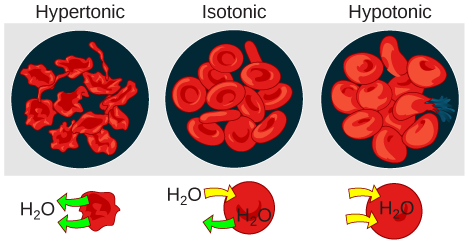# 5.9: Passive Transport - Tonicity

$$\newcommand{\vecs}{\overset { \rightharpoonup} {\mathbf{#1}} }$$ $$\newcommand{\vecd}{\overset{-\!-\!\rightharpoonup}{\vphantom{a}\smash {#1}}}$$$$\newcommand{\id}{\mathrm{id}}$$ $$\newcommand{\Span}{\mathrm{span}}$$ $$\newcommand{\kernel}{\mathrm{null}\,}$$ $$\newcommand{\range}{\mathrm{range}\,}$$ $$\newcommand{\RealPart}{\mathrm{Re}}$$ $$\newcommand{\ImaginaryPart}{\mathrm{Im}}$$ $$\newcommand{\Argument}{\mathrm{Arg}}$$ $$\newcommand{\norm}{\| #1 \|}$$ $$\newcommand{\inner}{\langle #1, #2 \rangle}$$ $$\newcommand{\Span}{\mathrm{span}}$$ $$\newcommand{\id}{\mathrm{id}}$$ $$\newcommand{\Span}{\mathrm{span}}$$ $$\newcommand{\kernel}{\mathrm{null}\,}$$ $$\newcommand{\range}{\mathrm{range}\,}$$ $$\newcommand{\RealPart}{\mathrm{Re}}$$ $$\newcommand{\ImaginaryPart}{\mathrm{Im}}$$ $$\newcommand{\Argument}{\mathrm{Arg}}$$ $$\newcommand{\norm}{\| #1 \|}$$ $$\newcommand{\inner}{\langle #1, #2 \rangle}$$ $$\newcommand{\Span}{\mathrm{span}}$$$$\newcommand{\AA}{\unicode[.8,0]{x212B}}$$

##### Learning Objectives
• Define tonicity and describe its relevance to osmosis

Tonicity describes how an extracellular solution can change the volume of a cell by affecting osmosis. A solution’s tonicity often directly correlates with the osmolarity of the solution. Osmolarity describes the total solute concentration of the solution. A solution with low osmolarity has a greater number of water molecules relative to the number of solute particles; a solution with high osmolarity has fewer water molecules with respect to solute particles. In a situation in which solutions of two different osmolarities are separated by a membrane permeable to water, though not to the solute, water will move from the side of the membrane with lower osmolarity (and more water) to the side with higher osmolarity (and less water). This effect makes sense if you remember that the solute cannot move across the membrane, and thus the only component in the system that can move—the water—moves along its own concentration gradient. An important distinction that concerns living systems is that osmolarity measures the number of particles (which may be molecules) in a solution. Therefore, a solution that is cloudy with cells may have a lower osmolarity than a solution that is clear if the second solution contains more dissolved molecules than there are cells.

## Hypotonic Solutions

Three terms—hypotonic, isotonic, and hypertonic—are used to relate the osmolarity of a cell to the osmolarity of the extracellular fluid that contains the cells. In a hypotonic situation, the extracellular fluid has lower osmolarity than the fluid inside the cell, and water enters the cell. (In living systems, the point of reference is always the cytoplasm, so the prefix hypo- means that the extracellular fluid has a lower concentration of solutes, or a lower osmolarity, than the cell cytoplasm. ) It also means that the extracellular fluid has a higher concentration of water in the solution than does the cell. In this situation, water will follow its concentration gradient and enter the cell, causing the cell to expand.Figure $$\PageIndex{1}$$: Changes in Cell Shape Due to Dissolved Solutes: Osmotic pressure changes the shape of red blood cells in hypertonic, isotonic, and hypotonic solutions.

## Hypertonic Solutions

As for a hypertonic solution, the prefix hyper- refers to the extracellular fluid having a higher osmolarity than the cell’s cytoplasm; therefore, the fluid contains less water than the cell does. Because the cell has a relatively higher concentration of water, water will leave the cell, and the cell will shrink.

## Isotonic Solutions

In an isotonic solution, the extracellular fluid has the same osmolarity as the cell. If the osmolarity of the cell matches that of the extracellular fluid, there will be no net movement of water into or out of the cell, although water will still move in and out.

Blood cells and plant cells in hypertonic, isotonic, and hypotonic solutions take on characteristic appearances. Cells in an isotonic solution retain their shape. Cells in a hypotonic solution swell as water enters the cell, and may burst if the concentration gradient is large enough between the inside and outside of the cell. Cells in a hypertonic solution shrink as water exits the cell, becoming shriveled.

## Key Points

• Osmolarity describes the total solute concentration of a solution; solutions with a low solute concentration have a low osmolarity, while those with a high osmolarity have a high solute concentration.
• Water moves from the side of the membrane with lower osmolarity (and more water) to the side with higher osmolarity (and less water).
• In a hypotonic solution, the extracellular fluid has a lower osmolarity than the fluid inside the cell; water enters the cell.
• In a hypertonic solution, the extracellular fluid has a higher osmolarity than the fluid inside the cell; water leaves the cell.
• In an isotonic solution, the extracellular fluid has the same osmolarity as the cell; there will be no net movement of water into or out of the cell.

## Key Terms

• osmolarity: The osmotic concentration of a solution, normally expressed as osmoles of solute per litre of solution.
• hypotonic: Having a lower osmotic pressure than another; a cell in this environment causes water to enter the cell, causing it to swell.
• hypertonic: having a greater osmotic pressure than another
• isotonic: having the same osmotic pressure

This page titled 5.9: Passive Transport - Tonicity is shared under a CC BY-SA 4.0 license and was authored, remixed, and/or curated by Boundless.